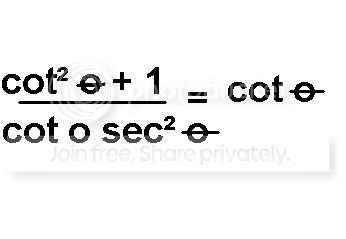XP Math - Forums - View Single Post - Proving: Fundamental Trigonometric Identities
 View Single Post01-18-2009   #2
MAS1Join Date: Dec 2008
Posts: 249Quote:
 Originally Posted by masturjohjiHello , could someone help me with this problem prove that:please explain them to me.
Start with the left side of the equation and make it look like the right side.

I will use x in place of theta.

1. (cot^2x + 1)/(cotxsec^2x) = cotx

2. ((cos^2x)/(sin^2x) + 1)/((cosx/sinx)(1/cos^2x)) = cotx

3. ((cos^2x + sin^2x)/sin^2x)/(1/(sinxcosx)) = cotx

4. (1/sin^2x)/(1/sinxcosx) = cotx

5. (1/sin^2x)(sinxcosx/1) = cotx

6. cosx/sinx = cotx

7. cotx = cotx

Explanations

1. Original equation
2. cotx = cosx/sinx so substitute for cotx on left side, and secx = 1/cosx so substitute on left side.
3. get common denominator for top of left side fraction, and cancel out cosx on bottom of left side fraction.
4. Substitute 1 = sin^2x + cos^2x
5. Change from division to multiplication.
6. Cancel out a sinx
7. definition of cotx = cosx/sinx

Hope this is understandable.